# Ellipse Parts

 Given an ellipse only, construct its major and minor axes, center, vertices, foci, and directrices.
 Begin by constructing a chord anywhere on the figure, and construct the midpoint of that chord.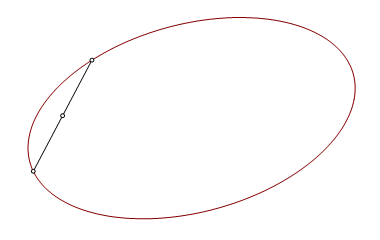Construct a second chord parallel to the first, and construct its midpoint. The line through the midpoints is an oblique axis of the ellipse.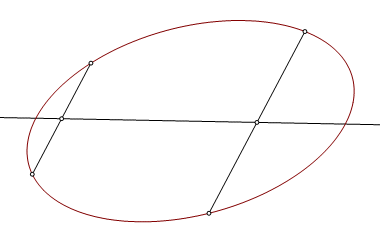The intersections of the oblique axis and the ellipse are not vertices (not in the usual sense), but they do have this useful propertery. The midpoint, O, of the chord is the center of the ellipse.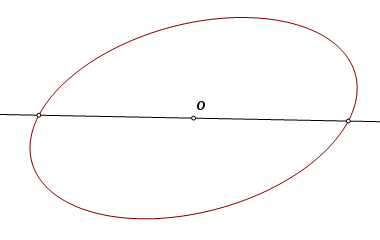Construct a circle centered on point O and intersecting the ellipse at four points. These are the vertices of an inscribed rectangle.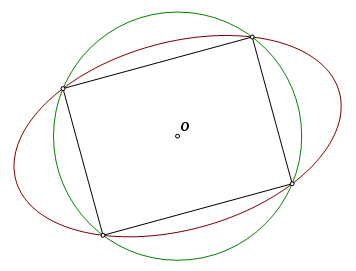The rectangle and the ellipse have the same axes of symmetry. Construct the lines through the midpoints of opposite sides of the rectangle. These are the axes of orthogonal symmetry of the ellipse. They intersect the ellipse at the four vertices, here labeled V1, V2, V3, and V4.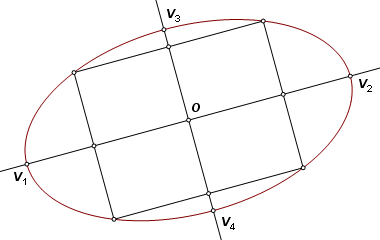From any point on the ellipse, the sum of the focus distances must be V1V2, the length of the major axis. Construct the circle with diameter V1V2. Translate it so that the image is centered on minor vertex V3. Let it intersect the major axis at F1 and F2. These are the foci because they are on the major axis and satisfy these two conditions: V3F1 + V3F2 = V1V2 V3F1 = V3F2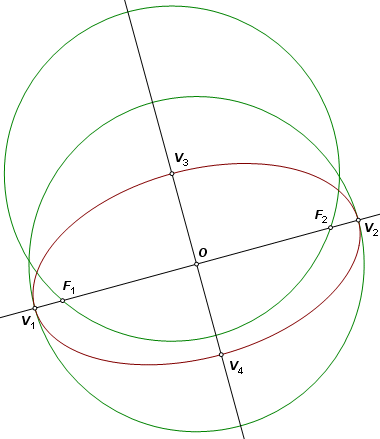Let K be the point where the major axis intersects the directrix corresponding to focus F1. Then KF1 must divide V1V2 harmonically. Equivalently, K is the inverse of F1 with respect to the circumcircle. To construct K, project F1 perpendicular to the major axis onto the circle, then tangent to the circle onto the major axis. The directrix is then constructed through K perpendicular to the major axis, and the other directrix is its reflection across the minor axis.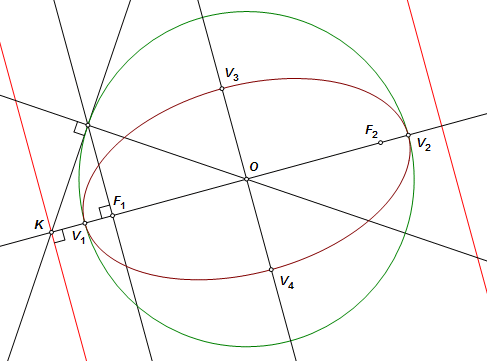The completed construction.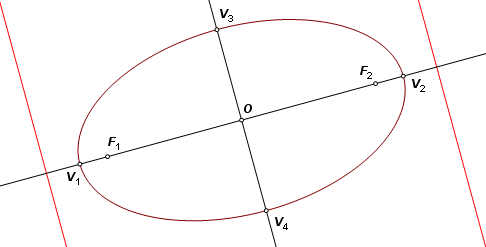Back to Conic Constructions Back to Whistler Alley Mathematics Last update: January 24, 2012 ... Paul Kunkel whistling@whistleralley.com For email to reach me, the word geometry must appear in the body of the message.